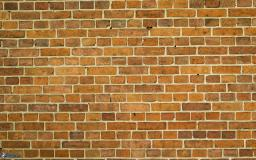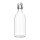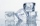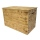# The wall

We have to build a cuboid wall with dimensions base 30 cm and 45 cm and height 3.25 meters. Calculate how many we need bricks if we spend 400 pieces of bricks to 1 m3 of wall?

Correct result:

n =  175.5

#### Solution:We would be pleased if you find an error in the word problem, spelling mistakes, or inaccuracies and send it to us. Thank you!Tips to related online calculators
Do you know the volume and unit volume, and want to convert volume units?

## Next similar math problems:

• The cubeThe cube has a surface of 600 cm2, what is its volume?
• Volume per timeHow long does fill take for a pump with a volume flow of 200 l per minute fill a cube-shaped tank up to 75% of its height if the length of the cube edge is 4 m?
• The woodenThe wooden block measures 12 cm, 24 cm, and 30 cm. Peter wants to cut it into several identical cubes. At least how many cubes can he get?
• Into boxHow many cubes with an edge of 2.5 cm fit into a box measuring 11.6 cm; 8.9 cm and 13.75 cm?
• Cargo spaceThe cargo space of the truck has the dimensions a = 4.2 m, b = 1.9 m, c = 8.1 dm. Calculate its volume.
• JansenJansen had 1.3 litres of fruit juice. He drank 0.5 litres during lunch. How many litres of fruit juice does he have left?
• AquariumHow many liters of water can fit in an aquarium measuring 30, 15 and 20 cm?
• Ice floeWhat is the volume of an ice floe weighing 326 kg? The density of ice is only 920 kg/m3.
• The bodyThe body has dimensions of 2m 2dm and 10 cm. It weighs 28 kg. What is its density?
• Cube V2SThe volume of the cube is 27 dm cubic. Calculate the surface of the cube.
• Brick wallWhat is the weight of a solid brick wall that is 30 cm wide, 4 m long and 2 m high? The density of the brick is 1500 kg per cubic meter.
• Thousand ballsWe have to create a thousand balls from a sphere with a diameter of 1 m. What will be their radius?
• Cylinder containerThe cylindrical container with a diameter of 1.8 m contains 2,000 liters of water. How high does the water reach?
• Aquarium heightHow high does the water in the aquarium reach, if there are 36 liters of water in it? The length of the aquarium is 60 cm and the width is 4 dm.
• Cuboid to cubeA cuboid with dimensions of 9 cm, 6 cm, and 4 cm has the same volume as a cube. Calculate the surface of this cube.
• Surface and volumeFind the surface and volume of a cuboid whose dimensions are 1 m, 50 cm, and 6 dm.
• Wooden boxThe block-shaped box was placed on the ground, leaving a rectangular print with dimensions of 3 m and 2 m. When flipped over to another wall, a print with dimensions of 0.5 m and 3 m remained in the sand. What is the volume of the wooden box?